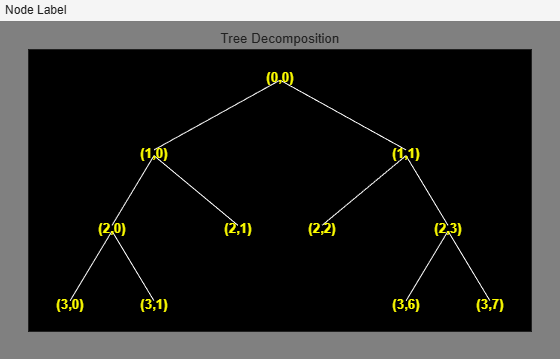# depo2ind

Node depth-position to node index

## Syntax

```N = depo2ind(ORD,[D P]) ```

## Description

`depo2ind` is a tree-management utility.

For a tree of order `ORD`, `N = depo2ind(ORD,[D P])` computes the indices `N` of the nodes whose depths and positions are encoded within `[D,P]`.

The nodes are numbered from left to right and from top to bottom. The root index is 0.

D and P are column vectors. The values of depths D and positions P must be such that D ≥0 and 0≤ PORDD-1.

Output indices N are such that 0 ≤ N < (ORDmax(D)-1)/ORD–1.

Note that for a column vector `X`, we have ```depo2ind(O,X) = X```.

## Examples

collapse all

Create a binary tree of depth 3. Plot the tree.

```ord = 2; t = ntree(ord,3); plot(t)```Merge the nodes of indices 4 and 5. Plot the new tree.

```t = nodejoin(t,5); t = nodejoin(t,4); figure plot(t)```List the depth-position of the tree nodes.

`aln_depo = allnodes(t,'deppos')`
```aln_depo = 11×2 0 0 1 0 1 1 2 0 2 1 2 2 2 3 3 0 3 1 3 6 ⋮ ```

Convert the depth-position to index.

`aln_ind = depo2ind(ord,aln_depo)`
```aln_ind = 11×1 0 1 2 3 4 5 6 7 8 13 ⋮ ```

## Version History

Introduced before R2006a